•深度学习
• 使用libsvm 实现MNIST数据库手写数字识别，正确率98.14. 包含matlab程序，libsvm库，以及60000张训练数据10000张测试数据
• MNIST手写Matlab程序，包含BP和CNN程序。不依赖任何库，包含MNIST数据，BP网络可达到98.3%的识别率，CNN可达到99%的识别率。CNN比较耗时，关于CNN的程序介绍：...
• 使用matlab实现的对MNIST手写数字进行识别，经测试，真实可用的。
• 1、Mnist_cnn.py 该脚本文件 用TensorFlow框架 实现CNN卷积神经网络处理Mnist手写数字识别数据集，准确率达到99.21%； 2、Mnist_cnn_tensorboard.py 该脚本文件在Mnist_cnn.py的基础上实现可视化。
• 利用matlab制作的手写数字识别系统、代码可读性强，结构清晰、GUI运行界面、在Matlab R2014b下编辑完成，有手写版，也可导入图片识别
• 60000*785矩阵，每一行为一个样本，其中28*28=784为灰度图片拉成的行向量，最后一列为对应数字标签
• CNN——卷积神经网络类数字识别matlab实现代码,原因是现在而与Matlab c++ / CUDA库前端比一个Matlab库。这个项目提供了matlab类卷积神经网络的实现。勒存这网络是由Yann和已经成功地使用在许多实际应用,如手写数字...
• CNN-MNIST手写识别程序和pdf,mnist手写数字识别原理,matlab源码.zip
• Mnist手写数据集的Matlab版本（训练数据及标签、测试数据及标签）; 其中，训练数据共60000个， 测试数据共10000个；更多详情见MNIST官网http://yann.lecun.com/exdb/mnist/
• 代码主要实现了对手写数字识别，可通过代码得到识别的错误率，
• 利用卷积神经网络方法编写的简单的CNN-MNIST手写识别程序
• 本文运用BP神经网络对MNIST手写数字字符进行识别。 BP神经网络是通过样本以及期望输出不断调整权值以达到训练的目的的算法。本文采用三层BP神经网络对MNIST字符进行训练，然后对提供的数据进行测试。 二.Content...

一.Summary
本文运用BP神经网络对MNIST手写数字字符进行识别。
BP神经网络是通过样本以及期望输出不断调整权值以达到训练的目的的算法。本文采用三层BP神经网络对MNIST字符进行训练，然后对提供的数据进行测试。

二.Content
1. 分析BP神经网络的基本原理：通过输入向量与权向量加权求和，得到一个下层神经元的输入，再加上偏置，经过激活函数计算，作下层神经元的输出。
2. 该文采用经典的三层神经网络为例，反向传播的过程如下：
先求出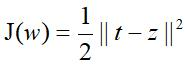,
其中J(w)为训练误差，t为输出端的期望输出值，z为实际输出值。
令w表示网络里所有的权值，根据J(w)调整w的大小：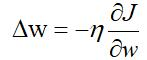，
继续的推导需要基于链式法则，具体过程可参考<模式分类>P237 6.3.1.最终得到隐含层到输出曾的权值更新规则：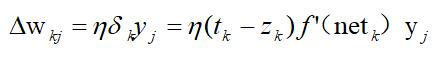输入层到隐含层权值的权值更新规则是：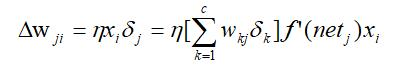具体符号含义都包含在<模式分类>中。
3. 具体训练过程如下：
①首先产生随机的权值w1,w2，生成偏置，初始化所用变量，设置神经网络层数及单元.
②进行前向传递算法，获得实际输出值z，选取激活函数为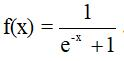③进行反向传播算法（BP），对权值w1,w2偏置值进行调整。
④迭代‘②③’过程以提高训练的准确率。
4. 测试并收集结果.
将测试集运用前向传递算法算出预测值，与实际期望预测值进行对比.
由于神经网络训练过程中随机生成权值，每次训练的结果具有随机性，因此重复文收集结果.
设置三层神经元，隐含层包含10个神经元，进行N次迭代，测试的准确率如下：
训练序号  准确率  1  0.9012  2  0.9000  3  0.8930  4  0.8860  5  0.8734
取平均值结果为：89.07%
5. 修改神经网络神经元数量和层数，对结果进行对比分析.
修改隐藏层神经元为20个，其他保持不变，测得平均准确率为：
91.70%
三.Conclusion
本文通过BP神经网路对MNIST手写数字字符库进行训练和识别，当三层神经网络迭代十次，隐含层包含10个神经元就可以达到89.07%的准确率，当隐含层包含20个神经元时，可以达到91.70%的识别准确率(对于10000条测试用例),可以发现在层数不变提高了BP神经网络2.95%的准确率。
附录：
MNIST字符库官方网站:
http://yann.lecun.com/exdb/mnist/
读取MNIST MATLAB代码引用（一位前辈的博客）：
https://blog.csdn.net/fuwenyan/article/details/53954615
可直接使用的读取的数据（github）：https://github.com/gszswork/MNIST_data_matlab
读取文件代码：
train_ima = loadMNISTImages(url);
%读取四个文件
sigmoid函数：
function output = sigmoid(x)
output =1./(1+exp(-x));
end
训练代码：
%三层神经网络
sam_sum = 60000;
input = 784;
output = 10;
hid = 20;
w1 = randn([input,hid]);
baocun = w1;
w2 = randn([hid,output]);
bias1 = zeros(hid,1);
bias2 = zeros(output,1);
rate1 = 0.005;
rate2 = 0.005;                %设置学习率

%用作三层神经网络的暂时存储变量
temp1 = zeros(hid,1);
net = temp1;
temp2 = zeros(output,1);
z = temp2;

%sigmoid函数 f = 1/(e^-x + 1)   求导为 f' = f*(1-f)
for num = 1:100
num
for i = 1:sam_sum

label = zeros(10,1);
label(train_lab(i)+1,1) = 1;

%forward
%此处选用784*1的train_ima矩阵，因此需要加(:,1)
temp1 = train_ima(:,i)'*w1 + bias1';
net = sigmoid(temp1);
%此处选用hid*1的隐藏层矩阵
temp2 = net*w2 + bias2';
z = sigmoid(temp2);
z = z';net = net';
%backward
error = label - z;
deltaZ = error.*z.*(1-z);
deltaNet = net.*(1-net).*(w2*deltaZ);
for j = 1:output
w2(:,j) = w2(:,j) + rate2*deltaZ(j).*net;
end
for j = 1:hid
w1(:,j) = w1(:,j) + rate1*deltaNet(j).*train_ima(:,i);
end
bias2 = bias2 + rate2*deltaZ;
bias1 = bias1 + rate1*deltaNet;

end
end
测试代码：
test_sum = 10000;
count = 0;
for i = 1:test_sum
temp1 = test_ima(:,i)'*w1 + bias1';
net = sigmoid(temp1);
%此处选用hid*1的隐藏层矩阵
temp2 = net*w2 + bias2';
z = sigmoid(temp2);

[maxn,inx] = max(z);
inx = inx -1;
if inx == test_lab(i)
count = count+1;
end
end
correctRate = count/test_sum

博主自己通过测试，以上代码能够实现准确率85%以上，可以通过添加迭代次数，修改学习率进一步改进准确率。
该代码应用博主本科《机器学习》一课实验，仅供大家学习参考，如涉及assignment等提交，请慎重选择参考程度。


展开全文• BP神经网络实现手写数字识别，使用matlab实现。内有测试数据及实验结果，非常适合入门者使用。绝对超值、值5分。0.99MB的文件下载该文档，你不会吃亏。
• 手写数字识别
1.X版本说明
此版本基于老师所给的MATLAB代码改写，并对隐藏层大小、数据读取方式进行修改，提高收敛速度以及准确率
一、数据处理
import scipy.io as scio
## import torch
import numpy as np
## import torchvision
## 使用GPU资源
## device = torch.device('cuda' if torch.cuda.is_available() else 'cpu')

1. 直接读取mat文件
path=''

## 查看数据类型
data

{'__header__': b'MATLAB 5.0 MAT-file, Platform: MACI64, Created on: Wed Nov 20 21:23:10 2019',
'__version__': '1.0',
'__globals__': [],
'test_ima': array([[0, 0, 0, ..., 0, 0, 0],
[0, 0, 0, ..., 0, 0, 0],
[0, 0, 0, ..., 0, 0, 0],
...,
[0, 0, 0, ..., 0, 0, 0],
[0, 0, 0, ..., 0, 0, 0],
[0, 0, 0, ..., 0, 0, 0]], dtype=uint8),
'test_lab': array([,
,
,
...,
,
,
], dtype=uint8),
'train_ima': array([[0, 0, 0, ..., 0, 0, 0],
[0, 0, 0, ..., 0, 0, 0],
[0, 0, 0, ..., 0, 0, 0],
...,
[0, 0, 0, ..., 0, 0, 0],
[0, 0, 0, ..., 0, 0, 0],
[0, 0, 0, ..., 0, 0, 0]], dtype=uint8),
'train_lab': array([,
,
,
...,
,
,
], dtype=uint8)}

2. 将数据集转换为train、valid、test
## 制作训练集
train_ima=np.array(data['train_ima'])
train_lab=np.array(data['train_lab'])
length=len(train_lab)
train_feature=train_ima[:,:int(length*0.8)]
train_label=train_lab[:int(length*0.8):]
## 制作测试集
valid_feature=train_ima[:,int(length*0.8):]
valid_label=train_lab[int(length*0.8):]
## 制作检验集
test_feature=np.array(data['test_ima'])
test_label=np.array(data['test_lab'])

train_sum=len(train_label)
valid_sum=len(valid_label)
test_sum=len(test_label)
## 查看大小，确保划分的正确性
print(train_sum)

48000

3. 对MATLAB代码进行复现
import numpy as np

## 初始化模型参数
label_num=10
num_inputs = 784
num_hidden = 20
num_outputs = 10

W1 = np.random.normal(0, 0.01, (num_inputs, num_hidden))
b1 = np.zeros((num_hidden,1))
W2 = np.random.normal(0, 0.01, (num_hidden, num_outputs))
b2 = np.zeros((num_outputs,1))
## 设置梯度运算
rate1 = 0.05;
rate2 = 0.05;                #置学习率

## 定义需要用到的函数
def sigmord(x):
output =1./(1+np.exp(-x))
return output

def forward(x,w,b):
y=np.dot(x.reshape(1,-1),w).T + b
return y

## 训练与检测
## 在这一部分中，针对原代码进行了两项修改：

## 用作三层神经网络的暂时存储变量
temp1 = np.zeros((num_hidden,1))
net = temp1
temp2 = np.zeros((num_outputs,1))
z = temp2

batch_size=720
epochs=1

for epoch in range(epochs):
print('epoch:',epoch)
for num in range(int(train_sum/batch_size)):
## 随机读取数据
rand=np.random.randint(0,train_sum-1,batch_size)
## 训练
for i in rand:
label = np.zeros((label_num,1))
label[int(train_label[i])]=1

## 前向计算
temp1=forward(train_feature[:,i],W1,b1)
#temp1=np.dot(train_feature[:,i].reshape(1,784),W1).T + b1
net = sigmord(temp1).T
temp2=forward(net,W2,b2)
#temp2 = np.dot(net , W2).T + b2
z = sigmord(temp2)

## 误差计算
error = label - z
deltaZ = (error*z*(1-z))
deltaNet =(net*(1-net)).T*np.dot(W2,deltaZ)

## 参数更新
for j in range(num_outputs):
W2[:,j] = W2[:,j] + rate2*deltaZ[j]*net
for j in range(num_hidden):
W1[:,j] = W1[:,j] + (rate1*deltaNet[j]*train_feature[:,i])
b2 = b2 + rate2*deltaZ
b1 = b1 + rate1*deltaNet

## 检测
valid_sum=60000*0.2
count = 0
if num%10==0:
for i in range(int(valid_sum)):
temp1=np.dot(valid_feature[:,i].reshape(1,784),W1).T + b1
net = sigmord(temp1).T

temp2 = np.dot(net , W2).T + b2
z = sigmord(temp2)

inx =np.argmax(z)
inx=inx
if inx == valid_label[i]:
count=count+1
correctRate=count/valid_sum
print('step: ',num,'acc：',correctRate)


epoch: 0
step:  0 acc： 0.27841666666666665
step:  10 acc： 0.8729166666666667
step:  20 acc： 0.8755
step:  30 acc： 0.8913333333333333
step:  40 acc： 0.8919166666666667
step:  50 acc： 0.8993333333333333
step:  60 acc： 0.89525

## test
count=0
for i in range(test_sum):
temp1=np.dot(test_feature[:,i].reshape(1,784),W1).T + b1
#print(temp1.shape)
net = sigmord(temp1).T
#print(net.shape)

temp2 = np.dot(net , W2).T + b2
z = sigmord(temp2)

inx =np.argmax(z)
inx=inx
if inx == test_label[i]:
count=count+1
correctRate=count/test_sum
print('the test\'s acc：',correctRate)

the test's acc： 0.9013

## 保存参数
import csv
file = open('mnist_4.0.csv','w',encoding='utf-8')
# 2. 基于文件对象构建 csv写入对象
csv_writer = csv.writer(file)
# 3. 构建列表头
csv_writer.writerow(["batch_size","epochs","w1","w2","b1","b2","num_hidden"])
csv_writer.writerow([512,10,W1,W2,b1,b2,num_hidden])
# 5. 关闭文件
file.close()

对个别参数进行了“调乱”，直接跑无法得到相应精度，自行完善
欢迎私信或评论区讨论

展开全文深度学习
• MATLAB–基于BP神经网络的手写数字识别 在干活的过程中整理下来的，希望对大家有帮助。 手写数字识别技术作为图像处理和模式识别中的研究热点，在大规模数据统计（如行业年检、人口普查等）、票据识别、财务报表、...
MATLAB–基于BP神经网络的手写数字识别
在干活的过程中整理下来的，希望对大家有帮助。 手写数字识别技术作为图像处理和模式识别中的研究热点，在大规模数据统计（如行业年检、人口普查等）、票据识别、财务报表、邮件分拣等方面有着广泛的应用。目前手写数字识别技术也有很多种实现方法，这里介绍与本章内容相关的一种基于BP神经网络的手写数字识别方法。该方法的基本思想来源于以下两方面：一是手写数字图片中黑色像素点和白色像素点之间的空间编排关系构成了我们看到的数字，也就是说图片像素点的空间排布和输出的识别结果之间存在着非线性的映射关系。二是BP神经网络刚好就能解决非线性映射的数学建模问题。如果对像素点空间排布与输出数字之间的关系进行学习、训练，建立有效的人工神经网络，就可对再次给出的手写数字图片进行识别，输出识别结果。 手写数字的识别就是利用计算机自动识别人手写在纸张上的阿拉伯数字，如果输入以下手写数字图片，则计算机输出对应识别结果为：0、1、2、3、4、5、6、7、8、9。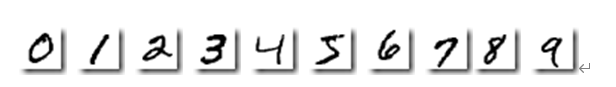进行手写数字识别时，先要对读取图片进行预处理，包括去噪、二值化等，再进行特征提取。特征提取的方法很多，这里采用一种简单的特征提取方法，即统计每个小区域中图像像素所占百分比作为特征数据。提取特征后再创建、训练BP神经网络模型，最后用测试数据进行测试。 要进行手写数字识别这个实际问题，第一步就是建立手写数字的模型。
1．手写数字识别非线性数学的模型建立
BP神经网络即误差反向传播神经网络，可解决有教师训练的非线性数学建模问题。模型的建立主要包括：BP神经网络的创建、BP神经网络的训练以及BP神经网络的测试三步。 创建BP神经网络前首先要确定网络的输入、输出。
（1）BP神经网络的输入、输出
BP神经网络的输入数据是手写数字样本经过预处理和特征提取后的数据。而为了BP神经网络的输入，我们肯定要先读入手写数字的样本，这里我们使用最通用的MNIST手写数字库，这个数据库直接在百度上就能下载到，该数据库包含0-9这10个数字的手写体，放在10个文件夹里，文件夹的名称对应存放的手写数字图片的数字，每个数字500张，每张图片的像素统一为28×28。 下面介绍用MATLAB程序实现读入手写数字的样本，这里注意，我将手写数字的样本放在和MATLAB 程序同根目录的文件夹里，文件夹的名称对应存放的手写数字图片的数字，也就是说当前文件夹如下图所示。画红线的文件夹就是我们要读入的手写数字的样本，以数字0为例，数据库中图片的名称如下图所示。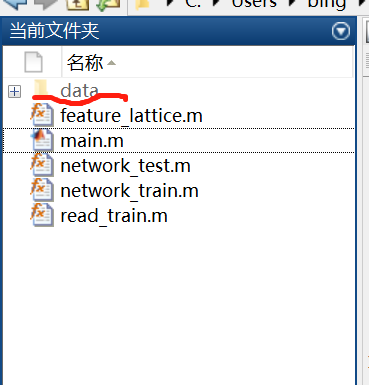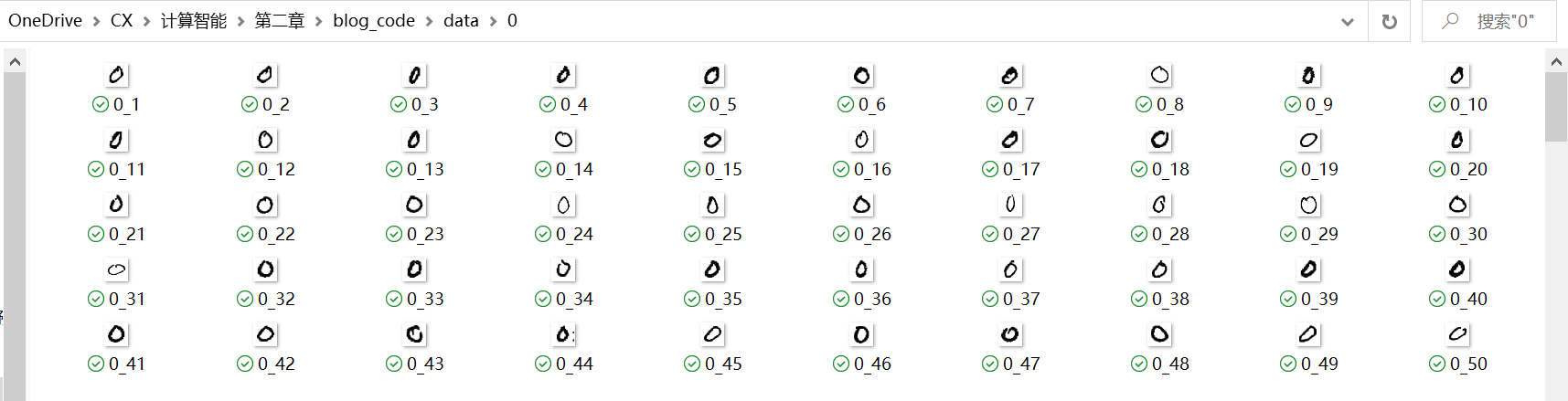这样就可以用在主程序中调用read_train子函数读入手写数字的样本了。
这里需要说明的是，我的MATLAB程序来自于： 版权声明：本文为博主原创文章，遵循 CC 4.0 BY-SA 版权协议，转载请附上原文出处链接和本声明。 本文链接：https://blog.csdn.net/zxm_jimin/article/details/87937652 ———————————————— 版权声明：本文为CSDN博主「zxm_」的原创文章，遵循 CC 4.0 BY-SA 版权协议，转载请附上原文出处链接及本声明。 原文链接：https://blog.csdn.net/zxm_jimin/article/details/87937652 这里主要介绍我在学习这段MALTLAB程序的时候，学到的内容。
main.m的MATLAB如下。
clc;
clear all;
close all;
%% 读取图像
root='./data';
%% 提取特征
img_feature=feature_lattice(img);
%% 构造标签
class=10;
numberpclass=500;
ann_label=zeros(class,numberpclass*class);
ann_data=img_feature;
for i=1:class
for j=numberpclass*(i-1)+1:numberpclass*i
ann_label(i,j)=1;
end
end

%% 选定训练集和测试集
k=rand(1,numberpclass*class);
[m,n]=sort(k);
ntraindata=4500;
ntestdata=500;
train_data=ann_data(:,n(1:ntraindata));
test_data=ann_data(:,n(ntraindata+1:numberpclass*class));
train_label=ann_label(:,n(1:ntraindata));
test_label=ann_label(:,n(ntraindata+1:numberpclass*class));
%% BP神经网络创建，训练和测试
net=network_train(train_data,train_label);
predict_label=network_test(test_data,net);
%% 正确率计算
[u,v]=find(test_label==1);
label=u';
error=label-predict_label;
accuracy=size(find(error==0),2)/size(label,2)

function [imglist] = read_train(root)
% ni为读取图片张数，n为文件夹数目
%========读取文件夹========%
out_Files = dir(root);%展开
tempind=0;
imglist=cell(0);
n=length(out_Files);
%========读取文件========%
for i = 1:n
if strcmp(out_Files(i).name,'.')|| strcmp(out_Files(i).name,'..')
else
rootpath=strcat(root,'/',out_Files(i).name);
in_filelist=dir(rootpath);
ni=length(in_filelist);
for j=1:ni
if strcmp(in_filelist(j).name,'.')|| strcmp(in_filelist(j).name,'..')|| strcmp(in_filelist(j).name,'Desktop_1.ini')|| strcmp(in_filelist(j).name,'Desktop_2.ini')
else
tempind=tempind+1;
end
end
end
end
end

在这个子程序的4行dir函数可以获得指定文件夹下的所有子文件夹和文件,并存放在文件结构体数组中。返回值out_Files是一个struct—结构体，我们可以在工作区查看它，如下图。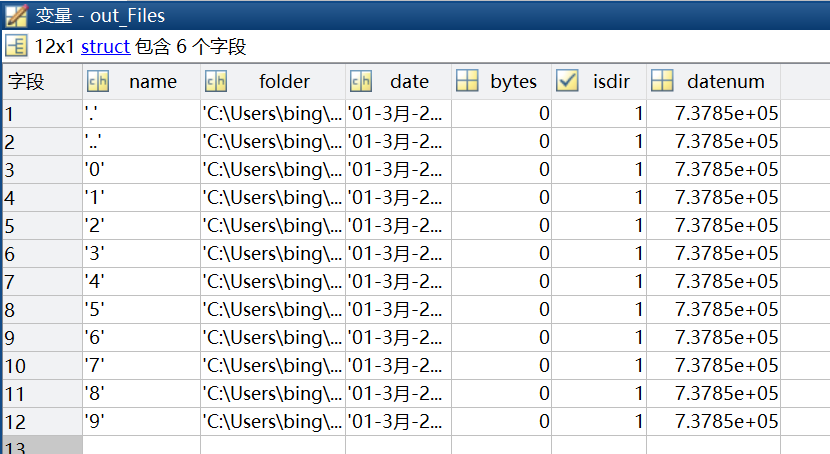在第10行函数strcmp（）用于做字符串的比较， 如调用格式为：TF=strcmp(s1,s2); 则如果字符串s1和s2是一致的，则返回值TF=1，否则，TF=0。 在第12行函数strcat（），用于横向连接字符串。如调用格式为：combinedStr= strcat(s1, s2, …, sN)，就是将数组 s1,s2,…,sN 水平地连接成单个字符串，并保存于变量combinedStr中。在这里就是讲文件夹的路径和文件夹名横向连接。 也就是说从10到13行，就是对于每一个文件夹中文件读入in_filelist这个结构体。而在n_filelist这个结构体中，比如对于”9”这个文件夹，每个图片的名称就是9_1，9_2,等等，从下边的一小块截图就可以看出来。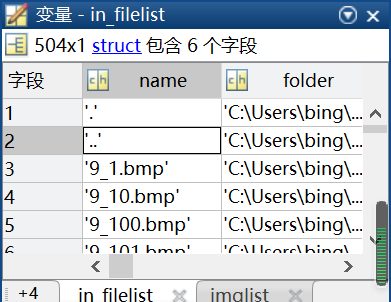从15到21行，同样用到了函数strcmp（）和函数strcat（），然后调用imread，也就是根据每个文件的路径及文件名，依次读入到imglist这个cell-元胞数组中，从而完成5000张图片的读入。
在我们这个例子预处理主要是图像的二值化，特征提取方法主要采用粗网格特征提取。粗网格特征提取方法是指将二值化后的图像大小统一为50×50，再等分成5×5个网格，每个网格包含100像素，然后依次统计每个网格中黑色像素点的数量，从而得到一个1×25的特征向量。 也就是说5000张手写数字图片的特征向量即为BP神经网络的输入（一个5000×25的矩阵），而其对应的真实数字为BP神经网络的输出（一个5000×1的向量）。 图像预处理及特征提取的MATLAB通过在主程序中调用feature_lattice子函数来实现，其MATLAB如下。
function feature = feature_lattice(img)
% 输入:黑底白字的二值图像。输出：25维的网格特征
% ======提取特征，转成5*5的特征矢量,把图像中每10*10的点进行划分相加，进行相加成一个点=====%
%======即统计每个小区域中图像象素所占百分比作为特征数据====%
for i=1:length(img);
bw2=im2bw(img{i},graythresh(img{i}));
bw_7050=imresize(bw2,[50,50]);
for cnt=1:5
for cnt2=1:5
Atemp=sum(bw_7050(((cnt*10-9):(cnt*10)),((cnt2*10-9):(cnt2*10))));%10*10box
lett((cnt-1)*5+cnt2)=sum(Atemp);
end
end
lett=((100-lett)/100);
lett=lett';
feature(:,i)=lett;
end

首先该子程序的第5行，在MATLAB中将图像转换为二值图像，调用MATLAB中的im2bw函数。 对于灰度图像，调用的格式如下。 bw=im2bw(I,level); level空着的话，默认是0.5。level一般使用graythresh函数来计算，graythresh函数是MATLAB中自带的，函数功能：使用最大类间方差法找到图片的一个合适的阈值–level， 第6行，调用imresize将图像大小统一为50×50。 接着，从8-13行，用嵌套的for循环语句，将图像分成5×5个网格，也就是说每个网格是10×10个像素点，然后统计每个网格中不为0的像素点的数量。 15行100-left就是每个网格中为0的像素点（黑色）的数量 第16行通过一个转置操作,保证每个图像的特征是一个列向量。 这样通过调用feature_lattice子函数，每一张图片都有1×25的特征向量。
提取完特征后，将数据打上标签，这段程序是主程序的10-18行。 因为数据一共10类，每类500张图片，先初始化一个用于存放标签的矩阵，这个矩阵大小就是10×(10×500)。 我用图画了一下这个存放标签的矩阵是什么样。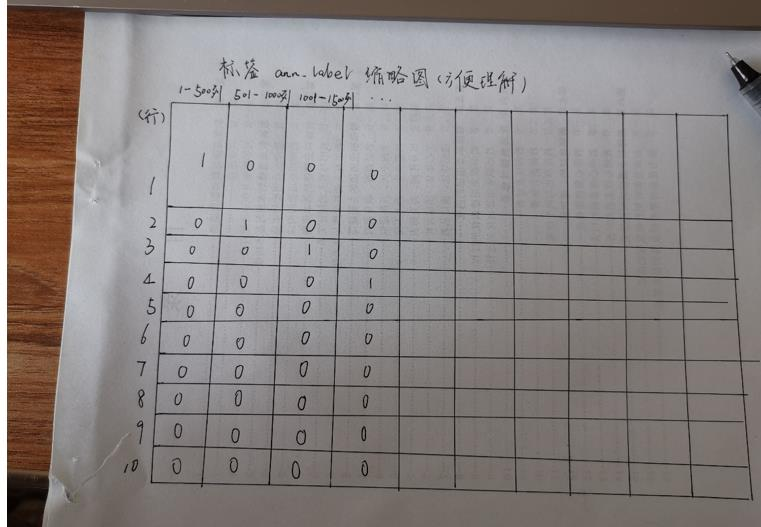如果一个图片的标签是[1;0;0;0;0;0;0;0;0;0],那么这张图片上的数字是1，如果标签是[0;1;0;0;0;0;0;0;0;0], 那么这张图片上的数字是2,以此类推。 因为我们数字图片是根据文件名读入的，这样图片上的数字、文件名和标签就都对应上了。
最后随机选取4500张图片作为训练样本，500张图片作为测试样本。MATLAB是主程序的21-28行。提取的特征在ann_data中，也就是BP神经网络的输入，标签在ann_label中，也就是BP神经网络的输入输出。这样，我们就将BP神经网络的输入、输出准备好了。就可以开始创建BP神经网络了。
（2）BP神经网络的创建
BP神经网络具有输入层、隐含层（隐藏层）和输出层的三层结构。其中，隐含层可以有一个或两个及以上，这里采用具有一个隐含层的基本BP网络模型，隐含层神经元个数选取25个，激励函数选取默认的Sigmoid函数，学习速率设为0.1，网络训练函数选取默认的Trainlm，网络目标误差设为0.001。随机抽取4500张图片提取的特征矩阵作为训练样本的输入，计算隐含层和输出层输出、误差，更新网络权值。当误差达到设定目标时，网络的学习过程就结束。在main.m中调用network_train子函数，其MATLAB程序如下。
function net = network_train(train_data,train_label )
% BP网络训练
% 初始化网络结构
layer=30;%隐含层个数
net=newff(train_data,train_label,layer);%创建网络
net.trainParam.epochs=10;%迭代次数
net.trainParam.lr=0.2;%学习率
net.trainParam.goal=0.001;%误差参数
net.trainFcn='trainrp';%训练方法
% 网络训练
net=train(net,train_data,train_label);
end

这样我们得到了训练好哒神经网络net。
（3）神经网络的测试
训练好神经网络之后，用随机抽取的500张手写数字图片对网络进行测试，输入特征向量，计算隐含层和输出层输出，得到最后测试的数据。同时计算每个数字的正确率和全体的正确率。在主程序中调用network_test子函数，其MATLAB程序如下。
function out = network_test(test_data,net)
% 输入：测试数据的特征和真值。输出：测试数据的label以及误差图
% BP网络预测
an=sim(net,test_data);
for i=1:length(test_data)
out(i)=find(an(:,i)==max(an(:,i)));
end
end

测试数据的标签存在了predict_label中，可以在工作区双击predict_label，打开查看。如下所示。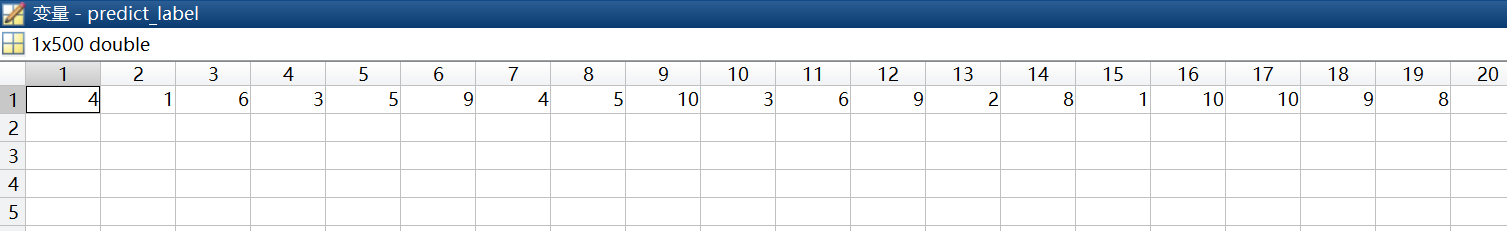正确率在命令行窗口可以看到。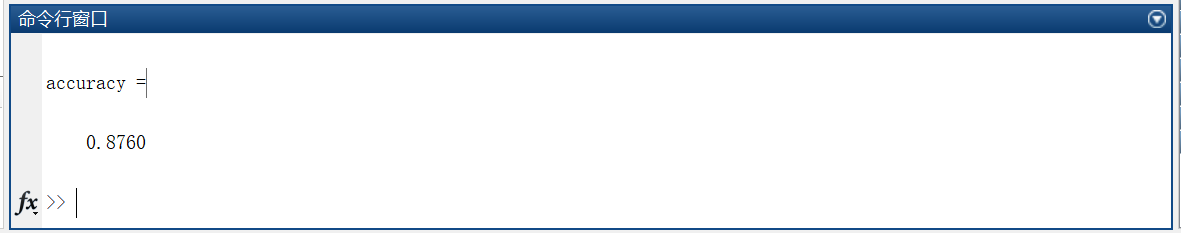另外，在网络训练的性能等可以点击下图中的按钮进行查看。这部分在上一篇介绍了，这里就不赘述了。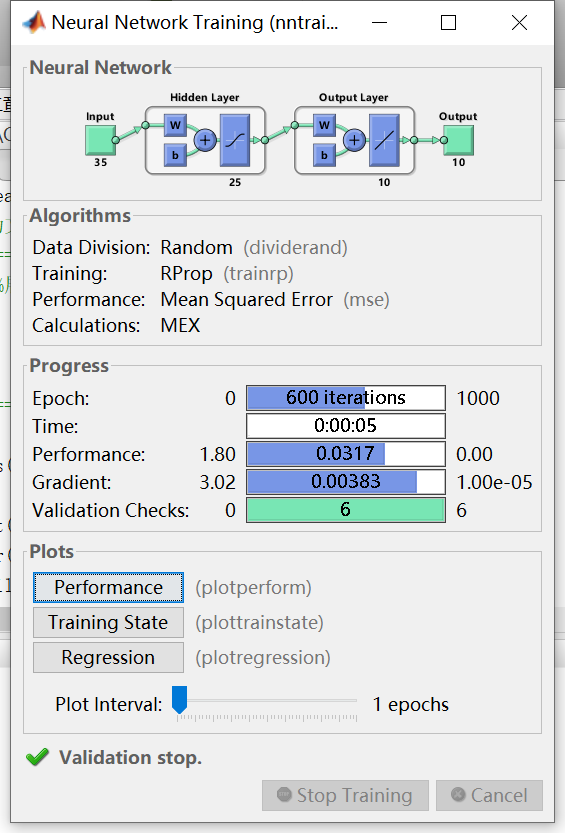手写数字的数据集，data，我存放在百度网盘里了。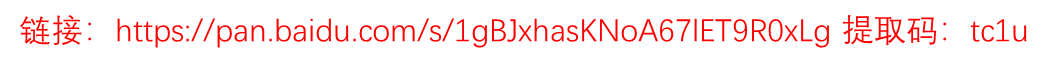展开全文神经网络
• 多层ELM进行MNIST手写字符分类MATLAB代码，直接运行.m程序，如果现实内存溢出，请改小隐藏节点个数。多层ELM
• MNIST手写数字识别Matlab代码：%Neural Networks Codes will be run on this part tic %%%%%%%%%%%%%%%%%%%%%%%%%%%%%%%%%%%%%%%%%%%%%%%%%%%%%%%%%%%%%%%%%%%%%%%%%%% clc clear all pic1=imread('8.png'); pic1=...
MNIST手写数字识别
Matlab代码：
%Neural Networks Codes will be run on this part
tic
%%%%%%%%%%%%%%%%%%%%%%%%%%%%%%%%%%%%%%%%%%%%%%%%%%%%%%%%%%%%%%%%%%%%%%%%%%%
clc
clear all
pic1=rgb2gray(pic1);
% pic=255-pic;
[a,b]=size(pic1);
for i=1:1:a
for j=1:1:b
if  pic1(i,j)==0
up=i;
break
end
end
end
for i=a:-1:1
for j=1:1:b
if  pic1(i,j)==0
down=i;
break
end
end
end
for j=1:1:b
for i=1:1:a
if  pic1(i,j)==0
left=j;
break
end
end
end
for j=b:-1:1
for i=1:1:a
if  pic1(i,j)==0
right=j;
break
end
end
end
pic=pic1(down:up,right:left);
imshow(pic)
pic=imresize(pic,[28 28]);
% size(pic);
pic1=1-double(reshape(pic,784,1))/255;
%%%%%%%%%%%%%%%%%%%%%%%%%%%%%%%%%%%%%%%%加载待识别图像
%%%%%%%%%%%%%%%%%%%%%%%%%%%%%%%%%%%%%%%%%%%%%%%%%%%%%%%%%%%%%%%%%%%%%%
label1=zeros(60000,10);
j=0;
for i=1:1:60000
j=label(i)+1;
label1(i,j)=1;
end
%%%%%%%%%%%%%%%%%%%%%%%%%%%%%%%%%%%%%%%%%%=训练样本
PR=minmax(image);
bpnet=newff(PR,[30 10],{'tansig', 'tansig'}, 'traingd', 'learngdm');
net.epoch=100;
net.trainParam.epochs=10;%允许最大训练步数
net.trainParam.goal=0.001; %训练目标最小误差0.001
net.trainParam.show=1; %每间隔100步显示一次训练结果
net.trainParam.lr=0.01; %学习速率
bpnet=train(bpnet,image,label1');
bpnet=sim(bpnet,pic1);
shuzi=find(bpnet==max(bpnet))-1
%%%%%%%%%%%%%%%%%%%%%%%%%%%%%%%%%%%%%%%%%%%%%%%%%%%%%%%%%%%%%%%%%%%%%%%%%%
toc
利用自己在写字板上写的字测试：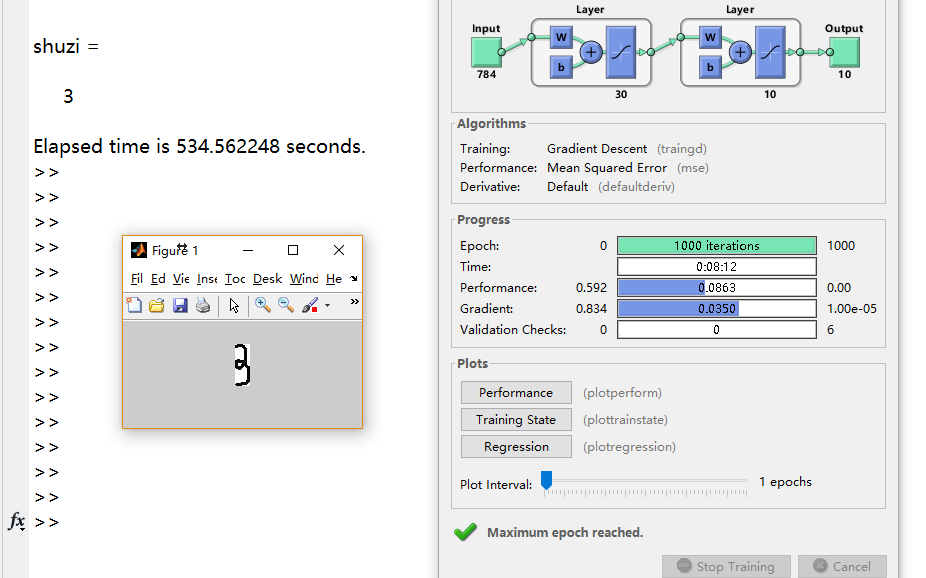如果不会下载MNIST 数据，可以访问：我的GitHub：
https://github.com/WeisongZhao/MNIST_Recognization
里面有不使用MATLAB工具箱的源代码
展开全文• 基于MATLAB编程实现手写数字识别算法，认识手写数字数据集，本实验用到的mnist手写数字数据集训练集包含60000张图片，测试集包含10000张图片。原始图片大小为28*28，去除边缘填充像素后的图片大小为20*20.本程序为...
• 系列博客是博主学习神经网络中相关的笔记和一些个人理解，仅为作者记录笔记之用，不免有很多细节不对之处...我们将⽤按照该教程相同的数据分割方法，将60,000个图像的 MNIST 训练集分成两个部分：⼀部分包含50,000个...
• 单特征 MNIST手写数字识别实现（matlab），采用粗网格特征进行学习识别，首先提取MNIST数据库60000个训练样本手进行特征提取，然后对10000个测试样本进行测试，matlab 实现
• 手写数字识别，所使用的是PCA主成分分析算法，内部有多个测试图片和PDF说明。
• matlab练习程序（神经网络识别mnist手写数据集）-附件资源
• MNIST手写Matlab程序，包含BP和CNN程序。不依赖任何库，包含MNIST数据，BP网络可达到98.3%的识别率，CNN可达到99%的识别率。CNN比较耗时，关于CNN的程序介绍：...卷积神经网络
• 本基于BP神经网络和卷积神经网络对手写数字识别进行研究，使用10000张已标注的大小为28*28的手写数字图片进行训练和测试，从所有图片中随机选出9000张作为训练样本对网络进行训练，另外1000张作为测试样本用于测试...
• MNIST手写识别 ReLU激活函数 规则化 识别率最高可达到97.5
• 代码及重要注释： # -*- coding: utf-8 -*- import tensorflow as tf from tensorflow.examples.tutorials.mnist import input_data ...mnist = input_data.read_data_sets("MNIST_data/", one_hot=True) stensorflow 深度学习 多层感知机
• MNIST手写数字包含60000张训练图片，10000张测试图片，图片大小为28×28，灰度图像，给出的是四个二进制存储的文件,分别为训练和测试的数据集和标签文件。假设读者已经明白所给链接博客的理论知识（不清楚可以参考更......

# mnist手写数字识别matlabmatlab 订阅